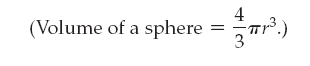×
Get Full Access to Introductory Chemistry - 5 Edition - Chapter 2 - Problem 125p
Get Full Access to Introductory Chemistry - 5 Edition - Chapter 2 - Problem 125p

×

# Answer: In 1999, scientists discovered a new class of black holes with masses 100 toISBN: 9780321910295 34

## Solution for problem 125P Chapter 2

Introductory Chemistry | 5th Edition

• Textbook Solutions
• 2901 Step-by-step solutions solved by professors and subject experts
• Get 24/7 help from StudySoup virtual teaching assistantsIntroductory Chemistry | 5th Edition

4 5 1 276 Reviews
21
4
Problem 125P

In 1999, scientists discovered a new class of black holes with masses 100 to 10,000 times the mass of our sun, but occupying less space than our moon. Suppose that one of these black holes has a mass of 1 × 103 suns and a radius equal to one-half the radius of our moon. What is its density in grams per cubic centimeter? The mass of the sun is 2.0 × 1030 kg, and the radius of the moon is 2.16 × 103 mi.

.Step-by-Step Solution:
Step 1 of 3

ARTH 2362.02 17 Jan. 2017 How to Read a Work of Art  Roger Van Der Weyden, Crucifixion Diptych, 1460,  Oil on Panel, 71”x73”  Northern Renaissance  Deposition: body taken off cross & laid in cultural  Diptych: 2 panels  Triptych: 3 panels ID Information o Artist: Roger Van Der Weyden o Title: Crucifixion...

Step 2 of 3

Step 3 of 3

##### ISBN: 9780321910295

The full step-by-step solution to problem: 125P from chapter: 2 was answered by , our top Chemistry solution expert on 05/06/17, 06:45PM. This textbook survival guide was created for the textbook: Introductory Chemistry, edition: 5. Since the solution to 125P from 2 chapter was answered, more than 886 students have viewed the full step-by-step answer. The answer to “In 1999, scientists discovered a new class of black holes with masses 100 to 10,000 times the mass of our sun, but occupying less space than our moon. Suppose that one of these black holes has a mass of 1 × 103 suns and a radius equal to one-half the radius of our moon. What is its density in grams per cubic centimeter? The mass of the sun is 2.0 × 1030 kg, and the radius of the moon is 2.16 × 103 mi..” is broken down into a number of easy to follow steps, and 84 words. This full solution covers the following key subjects: our, mass, radius, moon, Black. This expansive textbook survival guide covers 19 chapters, and 2045 solutions. Introductory Chemistry was written by and is associated to the ISBN: 9780321910295.

Unlock Textbook Solution